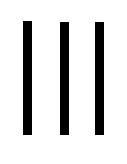# Quantity facts for kids

Kids Encyclopedia FactsMulticultural sign of quantity, refers to the simplest way of accounting - by sticks. Three sticks - the initial indicator of multiplicity as the first odd prime number.

Quantity or amount is how much of something there is that can be counted or measured.

## Counting

Whole numbers (1, 2, 3 ...) are used to count things. This can be done by pointing to each one. As things are pointed to, a number is said. Start with the number one. Each time another thing is pointed to, the next whole number is used. When the last thing in a group it pointed to, that number is the quantity of the group.

## Measuring

Measuring is done with a ruler or a machine. We put a ruler next to a thing to measure how long it is. We put things on a scale to measure their mass. Other machines are used to measure other quantities such as temperature, speed, electric current, and so on.

### Decimal numbers

When measuring things, a whole number might not be the best answer. A distance might be longer than 5 meters, but less than 6 meters. Meter sticks are marked off in parts of a meter. Decimals are formed by marking off a ruler in ten equal parts. Each part is a tenth. 5.2 meters is a little bit longer than 5 meters. 5.7 meters is a little bit shorter than 6 meters. Each of these tenths can be marked with ten smaller parts. The one hundred centimeters on a meter stick are done this way; by marking ten equal parts, then marking ten equal parts of each of those parts.

### Fraction numbers

Fractions are used when there are parts of a whole number. The dial on a machine may be marked with four parts between each whole number. A scale with a bag of potatoes may show five and three-quarters kilograms. This would be almost six kilograms.

### The words "amount" and "number"

People often use the word "amount" when they should say "number". The words "number" and "amount" should be used if a particular number could be put: e.g.

• A large amount of sand (because you cannot say "three thousand sands")
• A large number of people (because you could say there were 3547 people).Quantity Facts for Kids. Kiddle Encyclopedia.